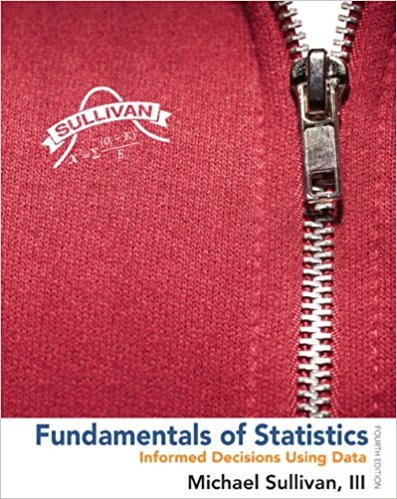×
×

# Solutions for Chapter Chapter 1.5: Fundamentals of Statistics 4th Edition## Full solutions for Fundamentals of Statistics | 4th Edition

ISBN: 9780321838704Solutions for Chapter Chapter 1.5

Solutions for Chapter Chapter 1.5
4 5 0 380 Reviews
24
1
##### ISBN: 9780321838704

This expansive textbook survival guide covers the following chapters and their solutions. This textbook survival guide was created for the textbook: Fundamentals of Statistics, edition: 4. Since 44 problems in chapter Chapter 1.5 have been answered, more than 284068 students have viewed full step-by-step solutions from this chapter. Fundamentals of Statistics was written by and is associated to the ISBN: 9780321838704. Chapter Chapter 1.5 includes 44 full step-by-step solutions.

Key Statistics Terms and definitions covered in this textbook
• Average run length, or ARL

The average number of samples taken in a process monitoring or inspection scheme until the scheme signals that the process is operating at a level different from the level in which it began.

• Bernoulli trials

Sequences of independent trials with only two outcomes, generally called “success” and “failure,” in which the probability of success remains constant.

• Box plot (or box and whisker plot)

A graphical display of data in which the box contains the middle 50% of the data (the interquartile range) with the median dividing it, and the whiskers extend to the smallest and largest values (or some deined lower and upper limits).

• Categorical data

Data consisting of counts or observations that can be classiied into categories. The categories may be descriptive.

• Center line

A horizontal line on a control chart at the value that estimates the mean of the statistic plotted on the chart. See Control chart.

• Components of variance

The individual components of the total variance that are attributable to speciic sources. This usually refers to the individual variance components arising from a random or mixed model analysis of variance.

• Conditional probability density function

The probability density function of the conditional probability distribution of a continuous random variable.

• Conditional probability distribution

The distribution of a random variable given that the random experiment produces an outcome in an event. The given event might specify values for one or more other random variables

• Confounding

When a factorial experiment is run in blocks and the blocks are too small to contain a complete replicate of the experiment, one can run a fraction of the replicate in each block, but this results in losing information on some effects. These effects are linked with or confounded with the blocks. In general, when two factors are varied such that their individual effects cannot be determined separately, their effects are said to be confounded.

• Conidence level

Another term for the conidence coeficient.

• Correlation matrix

A square matrix that contains the correlations among a set of random variables, say, XX X 1 2 k , ,…, . The main diagonal elements of the matrix are unity and the off-diagonal elements rij are the correlations between Xi and Xj .

• Counting techniques

Formulas used to determine the number of elements in sample spaces and events.

• Defects-per-unit control chart

See U chart

• Deining relation

A subset of effects in a fractional factorial design that deine the aliases in the design.

• Deming

W. Edwards Deming (1900–1993) was a leader in the use of statistical quality control.

• Deming’s 14 points.

A management philosophy promoted by W. Edwards Deming that emphasizes the importance of change and quality

• Discrete random variable

A random variable with a inite (or countably ininite) range.

• Dispersion

The amount of variability exhibited by data

• Error mean square

The error sum of squares divided by its number of degrees of freedom.

• Gamma random variable

A random variable that generalizes an Erlang random variable to noninteger values of the parameter r

×